• Call Now

1800-102-2727•

# Newton’s Third Law Of Motion: Explanation, Examples, Applications, Action-Reaction Pair

You probably know that when you throw a ball against a wall, the ball exerts a force on the wall. Likewise, the wall puts force on the ball as a result of which the ball bounces off the wall. Similarly, earth pulls you down with gravitational force. What you may not realize is you are also exerting an equal amount of force on the earth. This remarkable fact is a consequence of Newton's third law.

If you are an Avengers fan, you must have seen the Iron Man movie. You must have wondered how Tony Stark is able to fly when he wears the suit. This you can understand by Newton's third law of motion.

Newton's third law of motion states that to every action, there is an equal and opposite reaction. That means if a body A exerts a forceon another body B, then B exerts a force -on A, the two forces acting along the line joining the bodies.

• Magnitude of action reaction pair forces is equal.
• Direction of forces is opposite of each other.
• The pair of forces should act on two different bodies but not on the same bodies.

Examples:

1. Rocket is launched in space as it pushes the flumes of burnt fuel down on the ground.2. Swimmer pushes the water backwards and water exerts force on swimmer in forward direction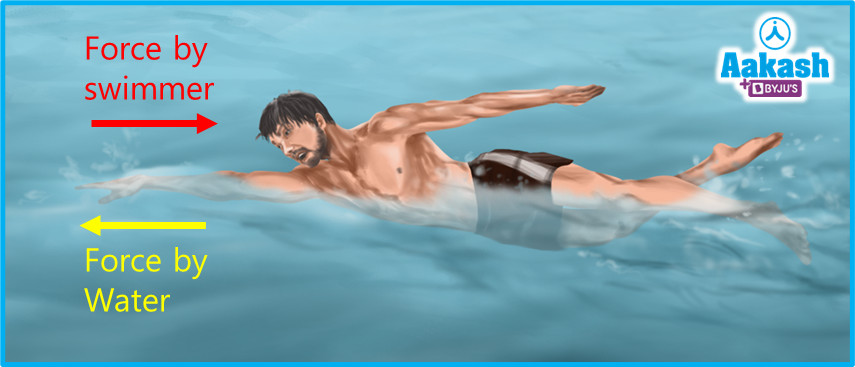## Action-Reaction Pair

From Newton's third law of motion,when there is force exerted by body A on body B, there is also a force exerted by body B on body A. These forces are equal in magnitude and act in opposite directions. Such a pair of forces is an action reaction pair.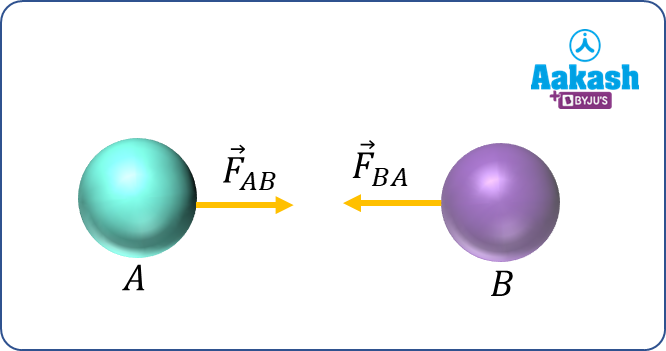Here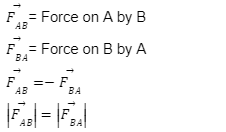## Conditions of Action-Reaction Pair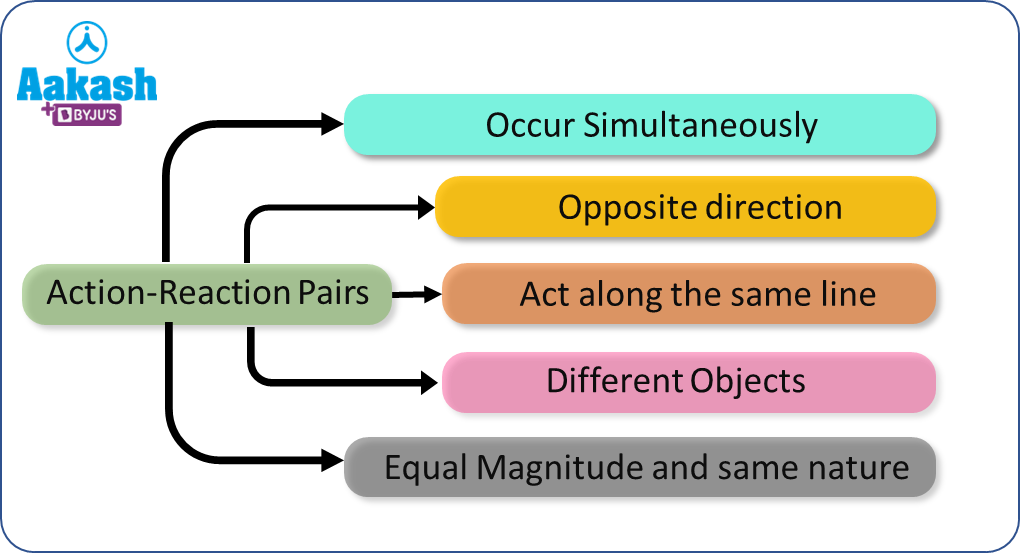## Application of Third Law

• Recoiling of gun: When a bullet is fired from a gun, it exerts forward force on the bullet and the bullet exerts equal and opposite force on the gun. Due to the high mass of the gun, it moves a little distance backward and gives a backward jerk to the shoulder of the gunman.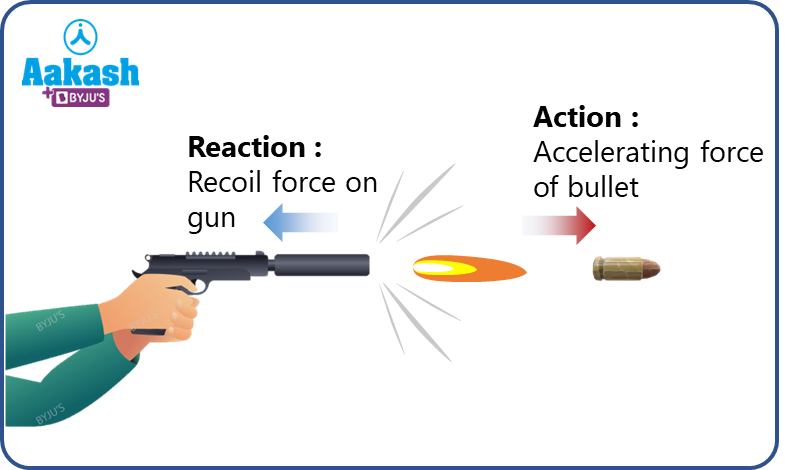• Walking: when we walk on the ground, our foot pushes the ground backward and in return the ground pushes our foot forward.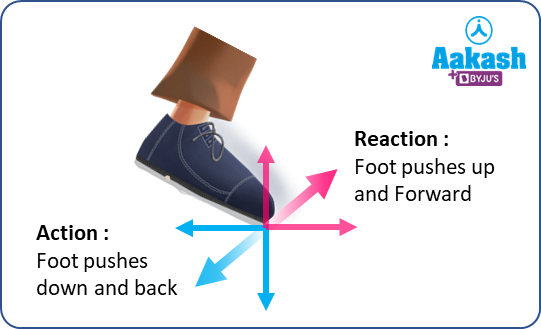• Jumping out from boat: When we start jumping out of a rowing boat, we push the boat backward with our feet. The boat exerts equal and opposite force on us in the forward direction which enables us to move forward.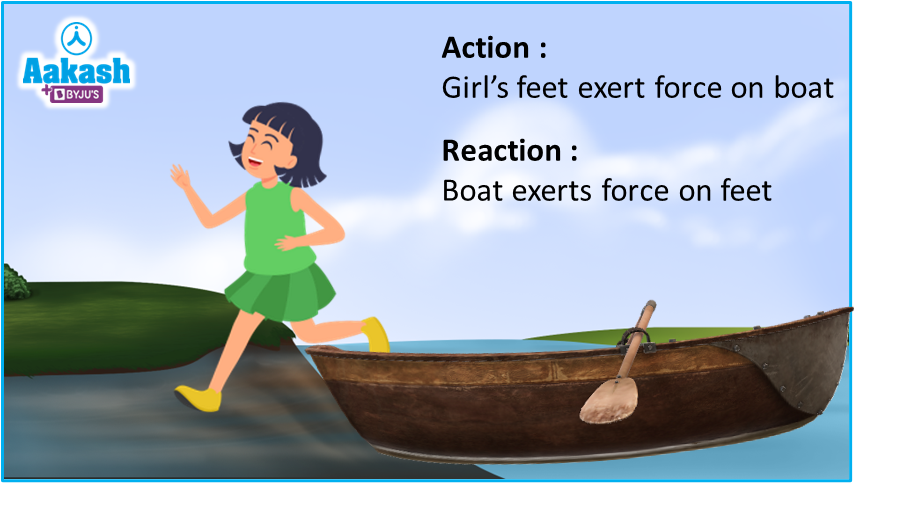## Third Law Contained in Second Law

Let’s try to deduce Newton’s third law using the second law of motion.

Now, consider a system of two bodies A and B. Also assume that there is no external force acting on the system.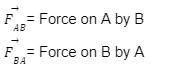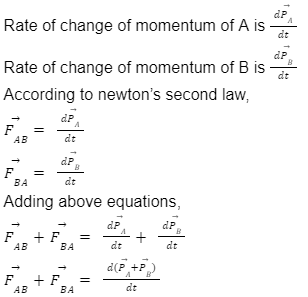As there is no external force on the system then there will be no change in the momentum because of no change in the velocity.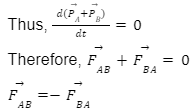Here we have proved Newton's third law using the second law of motion.

Video Explanation: Newton's third law

## Practice Problems of Newton's Third Law of Motion

Q1. If a bike collides with a fast moving truck, which object experiences greater acceleration?

Solution: According to Newton’s third law both experiences the same magnitude of force. As the mass of the bike is lesser compared to trucks so the bike experiences greater acceleration.

Q2. If a fly collides with the windshield of a fast moving bus, which object experiences impact force with larger magnitude?

Solution: Fly and bus both experience the same impact force according to the third law.

Q3. Two blocks of mass 4 Kg and 2 Kg are placed side by side on a smooth horizontal surface. Horizontal force of 20 N is applied on 4 Kg block. Find a normal reaction between the blocks?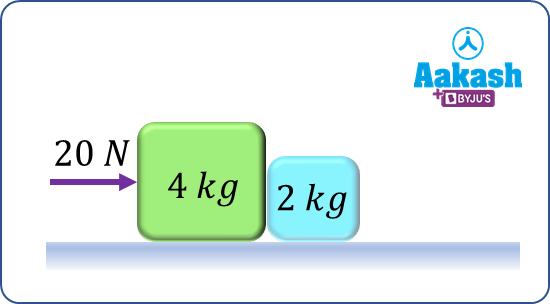Solution: Let’s take both block as system,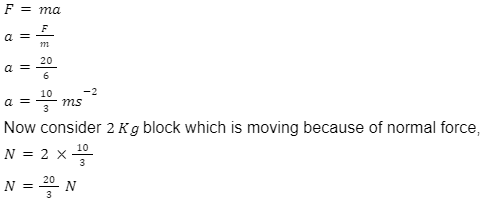Q4. A gun fires 6 bullets per second. The mass of each bullet is 10 g and the velocity of the bullet when it leaves the gun is 250 ms-1 the force required to hold the gun while firing is?

Solution:

Number of bullets = 6

Mass of bullet = 10 g=0.01 Kg

Velocity of bullet = 250 ms-1

Force required to hold the gun = number of bullets rate of change of momentum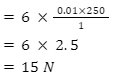## FAQs of Newton's Third Law of Motion

Question1. What is meant by an action-reaction pair?

Answer. The force exerted on an object is the action force, and the force experienced by the object as a consequence of Newton's third law is the reaction. They are equal in magnitude and opposite in direction.

Question2. Do weight and normal force acting on a block placed on a flat surface are action-reaction pairs?

Answer. No, because both forces are acting on the same body. Also weight and normal force are of different nature.

Question3. Give an example to explain Newton’s 3rd law of motion?

Answer. The swimmer while swimming pushes against the pool wall with his feet and in return accelerates (swims) in the direction opposite to that of his push.

Question4. Which of the laws can be derived from Newton’s second law of motion?

Answer. From Newton’s second law of motion we can derive the first and third law of motion.

## Related Topics to Newton's Third Law of Motion

 Newton’s First Law Of Motion, Inertia And Mass, Types Of Inertia, Practice Problems, FAQs Newton’s Second Law Of Motion- Its Mathematical Formulation, Derivation Of First Law Using Second Law, Impulse, Practice Problems, FAQs Free Body Diagram- System, Internal and External Forces, Steps to Draw FBD, Practice Problems, FAQs Resultant Force, Balanced Force, Unbalanced Force, Translational Equilibrium, Practice Problems, FAQsTalk to our expert
Resend OTP Timer =
By submitting up, I agree to receive all the Whatsapp communication on my registered number and Aakash terms and conditions and privacy policy